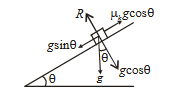#### A smooth block is released at rest on a  45o incline and then slides a distance. The time taken to slide  istime as much to slide on rough incline than on a smooth incline. The coefficient of friction is Option 1)Option 2)Option 3)Option 4)As we learnt in

Kinetic or Dynamic Friction -kinetic frictioncoefficient of kinetic friction

R = reaction

- whereindepends on the nature of surface in contact.

Component of down the plane

For smooth plane,

For rough plane,

Frictional retardation up the planeor

Putting

Correct option is 3.

Option 1)This is an incorrect option.

Option 2)This is an incorrect option.

Option 3)This is the correct option.

Option 4)This is an incorrect option.

#### prateek